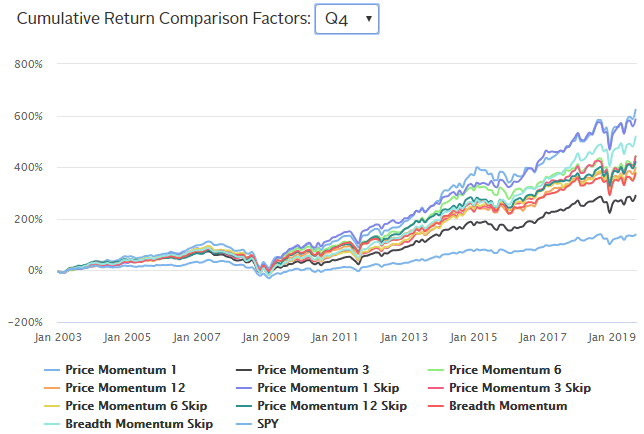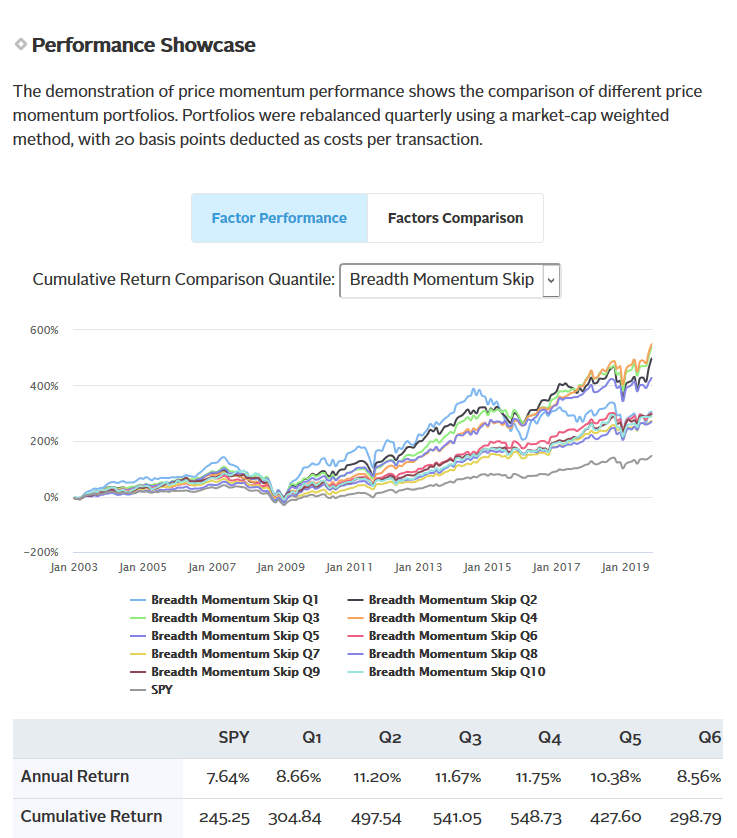Select Page

# The Kavout factor library – price and breadth momentum

Nov 28, 2019 | Factor Investment

When we launched our momentum factors, we broke them into four types: price momentum, risk-adjusted momentum, trend momentum, and idiosyncratic momentum. What are each of these?

• Price momentum is the rate of acceleration of a security’s price or volume, and a key factor for many investors. Momentum investing involves capitalizing on the continuance of an existing market trend. This includes going long on stocks showing upward-trending prices and shorting the respective assets with downward-trending prices.
• Risk-adjusted momentum is similar to price momentum, except this factor takes into account the level of risk involved. This is done primarily because of the popularity of the momentum factor during periods of high volatility.
• Trend momentum takes a rolling average of the price and return used in price momentum, looking to identify patterns of momentum among equities.
• Idiosyncratic momentum takes into account the three factors that explain a major part of the variation in average returns – market, size and value.

## How did we back test?

To demonstrate the performance of each momentum factor, we chose the largest 500 US equities by market capitalization, and then ranked them by its momentum factor. The universe was then divided into 10 quantiles of stocks. The top quantile (Q10) represents the stocks with the highest value for that given momentum factor, while the bottom quantile (Q1) represents the stocks with the lowest values of that momentum factor. The portfolios are rebalanced monthly and use a market-cap weighted method.

## Price momentum

Let’s take a closer look at price momentum. You can read more on Risk-adjusted momentum, Trend momentum, and Idiosyncratic momentum, and download free winning stock tickers ranked by momentum factors.

Typically, when referring to the momentum factor, price momentum is what most analysts will look at. Price momentum looks at the short, medium and long-term returns – considered at the one-month, three-month, six-month, and twelve-month time horizons.

The analyses also include skipping the most recent month due to the momentum factor’s short-term reversal effect – or the finding that previous winners (measured over the past month) do poorly the next month, while the previous losers (measured over the past month) do well the next month.

When we look closer at the Q4 portfolio, we see that the Price Momentum at one month has the highest cumulative return of all the price momentum factors – a cumulative return of 622.72%.

Interested in knowing which stocks are ranked the highest by Price momentum factors this month? Download free stock tickers by heading over to the Sample Data section.Breadth momentum is the weighted combination of one-month, three-month, six-month, and twelve-month price momentums. Below we’ve provided the performance charts for the portfolios for breadth momentum skipping the most recent month.

Based on the performance of the ten portfolios, portfolio Q4 has the highest cumulative return of 518.87% between January 2003 and September 2019. This significantly beats the benchmark cumulative return of 138.51%.

Head over to the Price Momentum Factors page, under Performance Showcase, select Breadth Momentum or Breadth Momentum Skip from the drop down window to see comparisons.

Interested in knowing which stocks are ranked the highest by Breadth momentum factors this month?  Download free stock tickers by heading over to the Sample Data section.